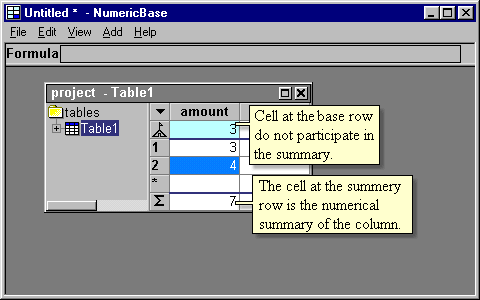# The summary row.

The summary row.
A cell at the summary row contains the numerical summary of all the cells at the same column.  The cell at the base row do not participate in the summary.Screenshot: the summary row.

Overriding the default behavior of the summary row .
If you want a calculation other than the numerical summary, you can enter a different formula. The following  screenshot uses the avg function to calculate the average rather than the summary.Screenshot: using average.